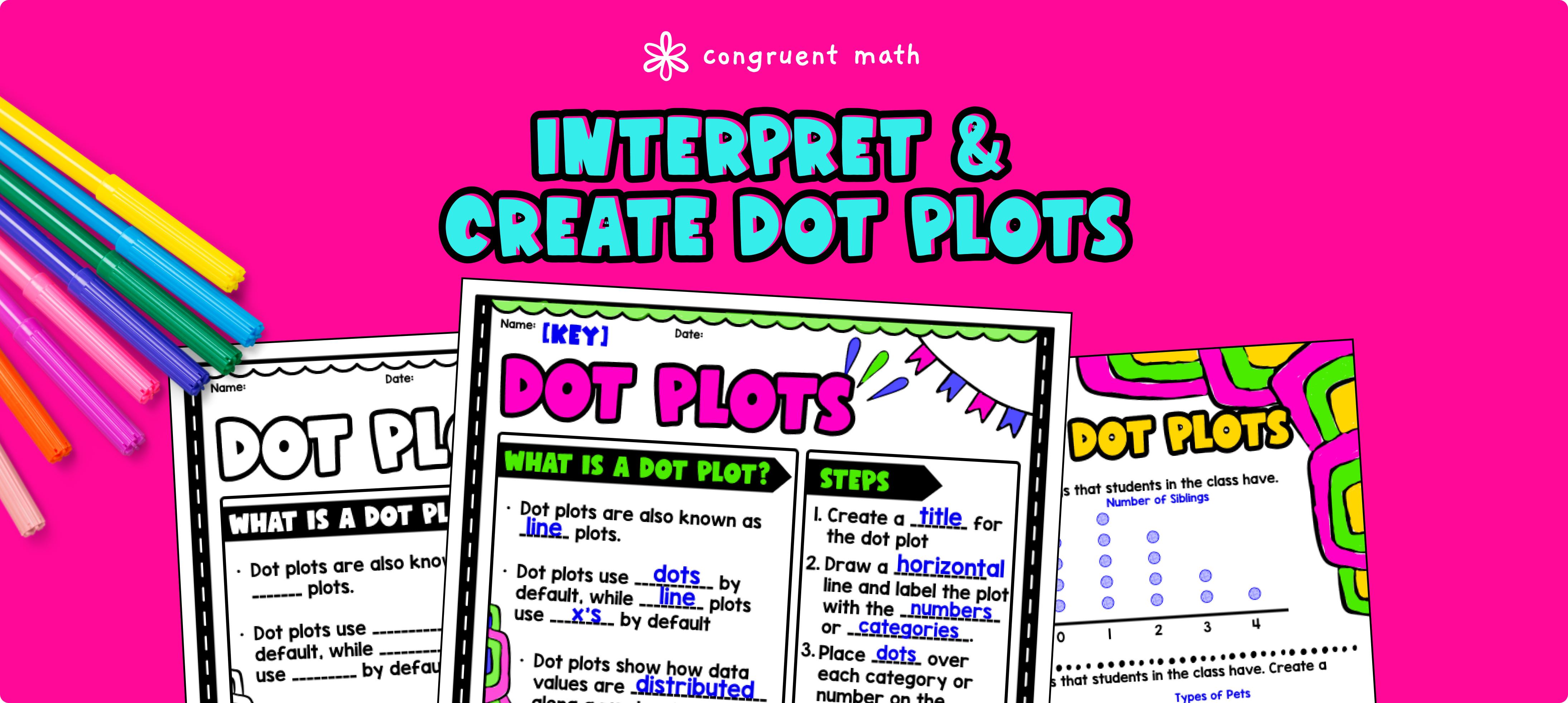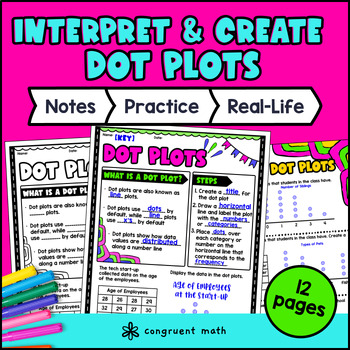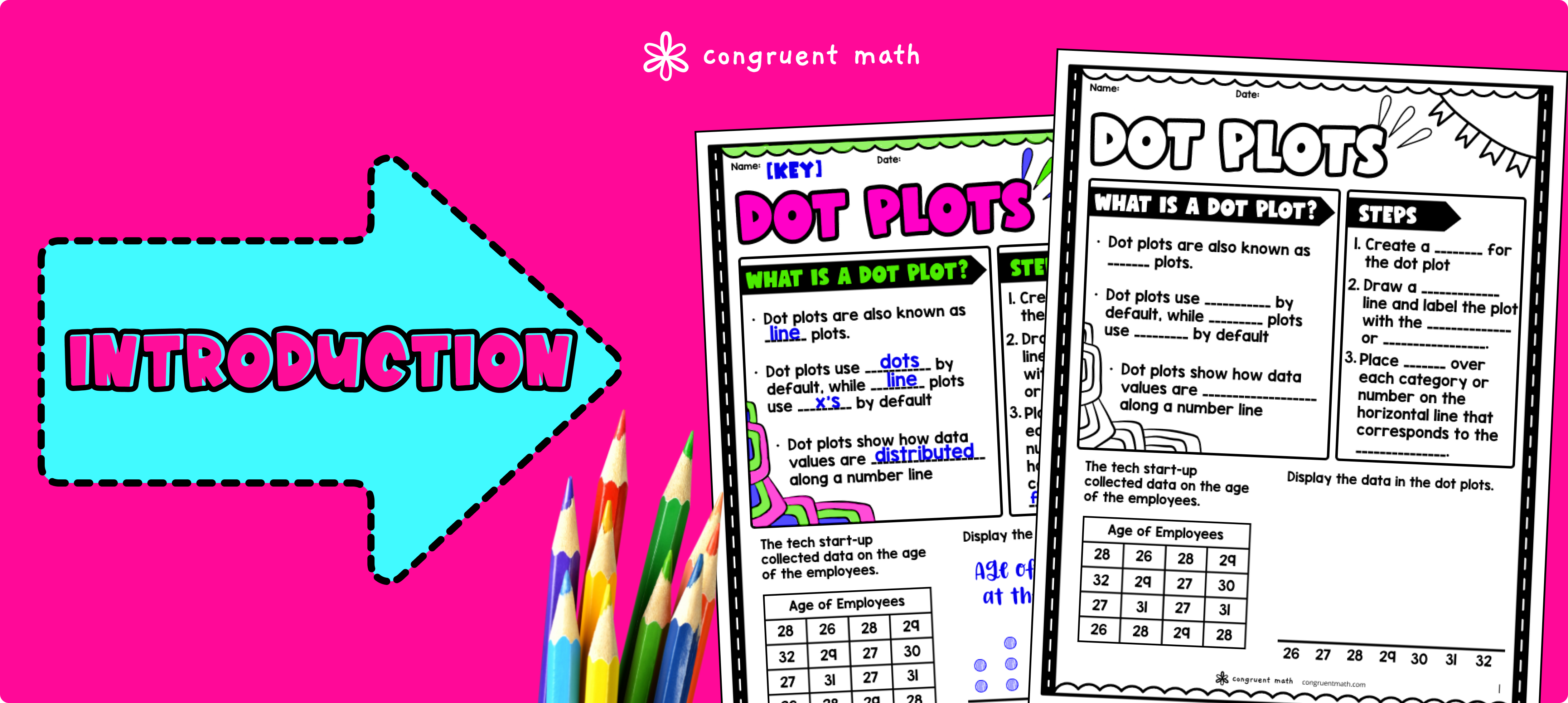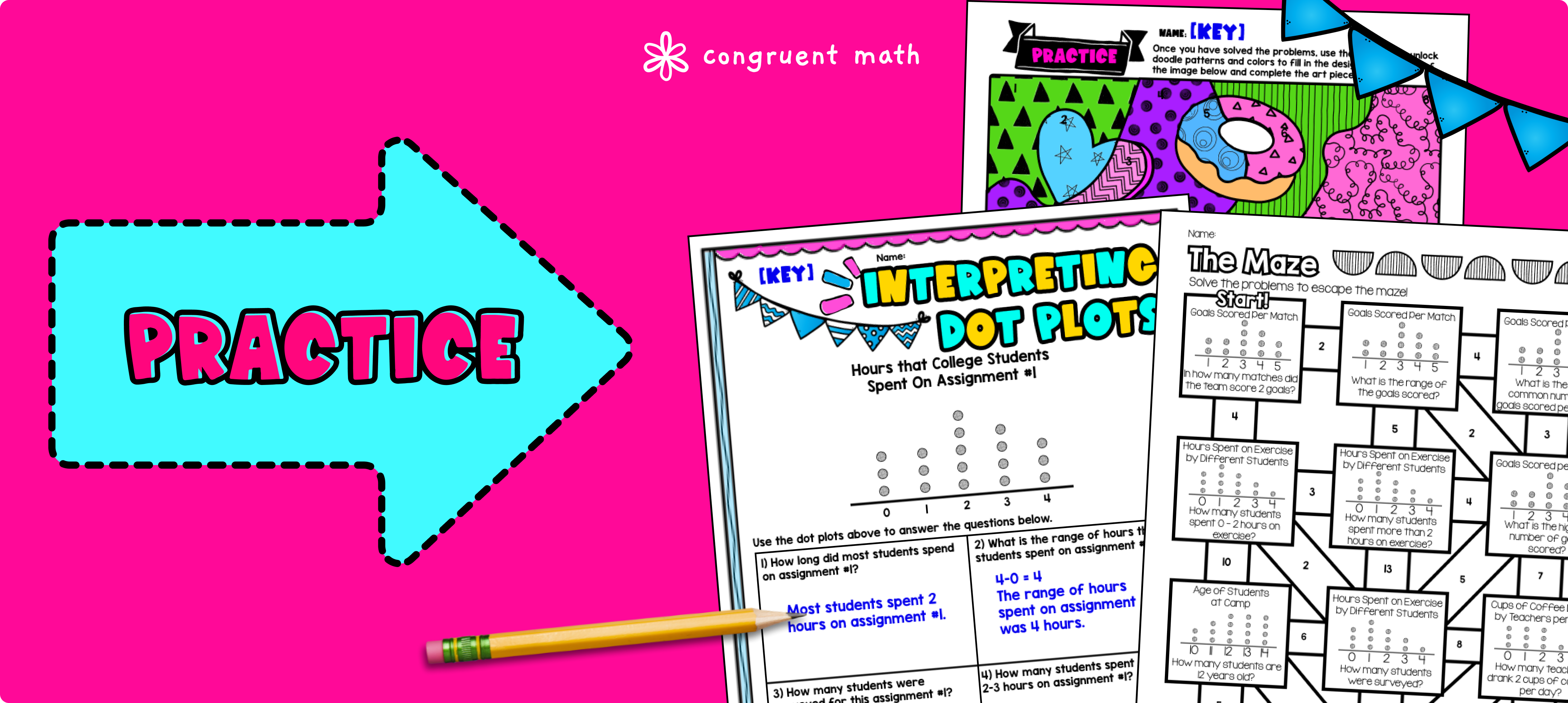# Construct & Interpret Dot Plots Lesson PlanPin This

## Overview

Ever wondered how to teach dot plots in an engaging way to your 6th grade students? In this lesson plan, students will learn how to construct and interpret dot plots and their real-life applications. Through artistic, interactive guided notes, check for understanding, a maze worksheet, and a doodle & color by number activity, students will gain a comprehensive understanding of dot plots.

The lesson culminates with a real-life example that explores how coaches can use dot plots to monitor the performance of their athletes in a variety of ways, such as identify star performers or tracking progress over time.

## Get the Lesson Materials\$4.25

## Learning Objectives

After this lesson, students will be able to:

• Construct and interpret dot plots

• Understand the real-life applications of dot plots

• Apply knowledge of dot plots to analyze data

## Prerequisites

Before this lesson, students should be familiar with:

• Basic understanding of how to label x-axis and y-axis of graphs

• Basic math skills, including addition, subtraction, and division of whole numbers

• Dot plot

• Frequency

• Interval

• Categories

## Procedure

### IntroductionPin This

As a hook, ask students why coaches might use dot plots to track the performance of their athletes. Refer to the last page of the guided notes as well as the FAQs below for ideas.

Use the guided notes to introduce the concept of dot plots. Walk through the key examples of of constructing and interpreting dot plots in page 2 and 3 of the guided notes. Refer to the FAQ below for a walk through on this, as well as ideas on how to respond to common student questions.

Have students work on the rest of the problems pertaining to the practice sheets (pg. 2 and 3). You can put the students together in small groups or pairs so they can learn from each other. Then, call on students to talk through their answers, potentially on the whiteboard or projector. Based on student responses, reteach concepts that students need extra help with.

### PracticePin This

Have students practice constructing and interpreting dot plots using the Maze worksheet from the lesson plan. Walk around to answer student questions.

Fast finishers can dive into the Doodle & Color by Number activity for extra practice. You can assign it as homework for the remainder of the class.

### Real-Life ApplicationPin This

Bring the class back together, and introduce the concept of sports statistics as a real-world application of dot plots. Coaches can use dot plots to monitor the performance of their athletes in a variety of ways, such as identifying star performers or tracking progress over time.

Refer to the FAQ section of the lesson plan for more ideas on how to teach it!

## Extensions

If you're looking for digital practice for dot plots, try the Pixel Art activities in Google Sheets. Every answer is automatically checked, and correct answers unlock parts of a mystery picture. It’s incredibly fun, and a powerful tool for differentiation.

Here's one activity to explore: Dot Plots, Histograms, and Stem-and-Leaf Plots 3 Pixel Art